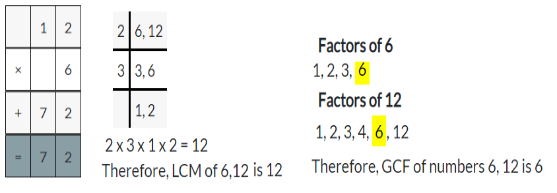# Math in Focus Grade 6 Chapter 1 Lesson 1.3 Answer Key Common Factors and Multiples

Go through the Math in Focus Grade 6 Workbook Answer Key Chapter 1 Lesson 1.3 Common Factors and Multiples to finish your assignments.

## Math in Focus Grade 6 Course 1 A Chapter 1 Lesson 1.3 Answer Key Common Factors and Multiples

### Math in Focus Grade 6 Chapter 1 Lesson 1.3 Guided Practice Answer Key

Complete.

Use the lists of factors on the right to find the common factors of 10 and 28.

Question 1.
The factors of 1o are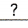,,, and.
The factors of 28 are,,,,and.
The common factors of 10 and 28 areand.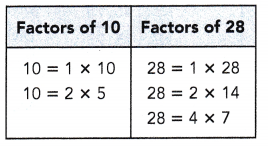The factors of 10are 1, 2, 5, 10
The factors of 28 are 1, 2, 4, 7, 4, 28
The common factors are 1 and 2.

Find the common factors of each pair of numbers.

Question 2.
16 and 24
1, 2 and 4.

Explanation:
The factors of 16 are 1, 2, 4, 8, 16
The factors of 24 are 1, 2, 3, 4, 6, 12, 24
The common factors of 16 and 24 are 1, 2 and 4.

Question 3.
27 and 35
1

Explanation:
The factors of 27 are 1, 3, 9, 27
The factors of 35 are 1, 5, 7, 35
The common factors of 27 and 35 is 1.

Question 4.
36 and 50
1 and 2.

Explanation:
The factors of 36 are 1, 2, 3, 4, 6, 9, 12, 18, 36
The factors of 50 are 1, 2, 5, 10, 25, 50
The common factors of 36 and 50 are 1 and 2.

Question 5.
40 and 54
1 and 2.

Explanation:
The factors of 40 are 1, 2, 4, 5, 8, 10, 20, 40
The factors of 54 are 1, 2, 3, 6, 9, 18, 27, 54.
The common factors of 40 and 54 are 1 and 2.

Complete.

Question 6.
Find the greatest common factor of 20 and 32.
Method 1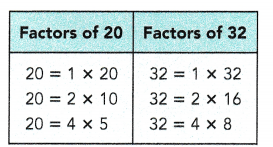The factors of 20 are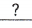,,,,, and.
The factors of 32 are,,,,, andThe common factors of 20 and 32 are,, and.
The greatest common factor of 20 and 32 is.

Method 2
By prime factorization,
20 = 2 •32 = 2 •Greatest common factor = 2 •=The greatest common factor of 20 and 32 is.

Method 3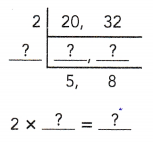The greatest common factor of 20 and 32 is.

Find the greatest common factor of each pair of numbers.

Question 7.
15 and 27
3

Explanation:
The factors of 15 = 1, 3, 5, 15
The factors of 27 = 1, 3, 9, 27
The common factors of 15 and  27 are 1 and 3
The greatest common factor of 15 and 27 is 3.

Question 8.
36 and 54
18

Explanation:
The factors of 36 are 1, 2, 3, 4, 6, 9, 12, 18, 36
The factors of 54 are  1, 2, 3, 6, 9, 18, 27, 54
The common factors of 36 and 54 are 1, 3, 6, 9, 18
The greatest common factor of 36 and 54 is 18.

Question 9.
48 and 72
24

Explanation:
The factors of 48 are 1, 2, 3, 4, 6, 8, 12, 16, 24, 48
The factors of 72 are 1, 2, 3, 4, 6, 8, 12, 16, 24, 36, 72
The common factors of 48 and 72 are 1, 2, 3, 4, 6, 8, 12, 16, 24
The greatest common factor of 48 and 72 is 24.

Question 10.
40 and 100
20

Explanation:
The factors of 40 are 1, 2, 4, 5, 8, 10, 20, 40
The factors of 100 are 1, 2, 4, 5, 10, 20, 25, 50, 100
The common factors of 40 and 100 are 1, 2, 4, 5, 10, 20.
The greatest common factors of 40 and 100 is 20.

Hands-On Activity

Find The Common Factors And The Greatest Common Factor Of Two Numbers

Materials:

• number cards (from 10 to 100)
• factor cards (2s, 3s, 5s, and 7s)

Work in pairs.

Step 1.
Shuffle the number cards and place them face down on a flat surface. Give half the factor cards to each player.

Step 2.
Each player turns over a number card and uses his or her factor cards to show the prime factorization of the number.

Step 3.
The first player states the greatest common factor (GCF) of the two numbers. If the player is correct, the player keeps the two number cards. Players reuse the factor cards on each turn.
Example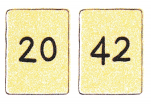Step 4.
Two new number cards are turned over and the process repeats. This time the second player gets to state the GCF.
Step 5.
Play continues until all number cards have been used. The player with more number cards is the winner.

Use the greatest common factor with the distributive property.

Express 12 + 20 as a product of the greatest common factor of the numbers and another sum.

First find the greatest common factor of the two numbers.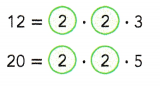Greatest common factor of 12 and 20 = 2 • 2
= 4
Then write the sum a different way. You know that
12 = 4 • 3 20 = 4 • 5
So, 12 + 20 = 4 • 3 + 4 • 5
= 4(3 + 5)
The distributive property says that:
4(3 + 5) = 4 • 3 + 4 • 5The number lines below show you that 12 + 20 and 4(3 + 5) represent the same number.
Notice that either way, you “end up” at the same place on the number line.
12 + 20 is a jump of 12 plus a jump of 20.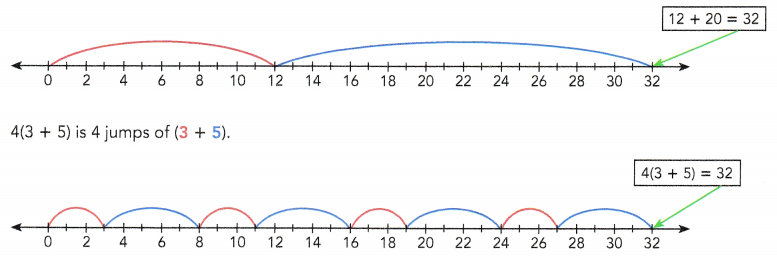Complete.

Question 11.
Express 18 + 45 as a product of the greatest common factor of the numbers and another sum.
By prime factorization,
18 = 2 •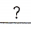45 = 3 •Greatest common factor of 18 and 45 ==18 + 45 =+=(+)

Express the sum of each pair of numbers as a product of the greatest common factor of the numbers and another sum.

Question 12.
35 + 91
7( 5 + 13 )

Explanation:
By prime factorization,
35 = 5 x 7, 91 = 7 x 13
Greatest common factor of 35 and 91 = 7
35 + 91 = 5 . 7 + 13 . 7
= 7(5+ 13)
35 + 91 = 7(5 + 13).

Question 13.
60 + 85
5 ( 12 + 17 )

Explanation:
By prime factorization,
60 = 2 x 2 x 3 x 5, 85 = 5 x 17
Greatest common factor of 60and 85 = 5
60 + 85 = 5 . 12 + 5 . 17
= 5( 12 + 17 )
60 + 85 = 5( 12 + 17 ).

Question 14.
24 + 64
8( 3 + 8 )

Explanation:
By prime factorization,
24 = 2 x 2 x 2 x 3, 64 = 2 x 2 x 2 x 2 x 2 x 2
Greatest common factor of 2 4and 64 = 2 . 2 . 2 = 8
24 + 64 = 8 . 3 + 8 . 8
= 8( 3 + 8)
24 + 64 = 8( 3 + 8 )

Complete.

Question 15.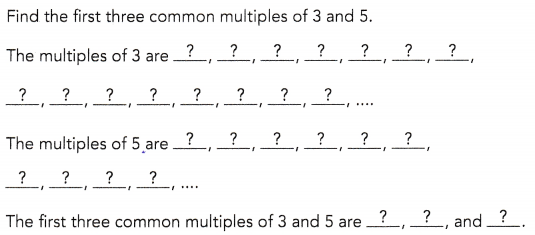15, 30 and 45

Explanation:
The multiples of 3 are 3, 6, 9, 12, 15, 18, 21, 24, 27, 30, 33, 36, 39, 42, 45
The multiples of 5 are 5, 10, 15, 20, 25, 30, 35, 40, 45
The first three common multiples of 3 and 5 are 15, 30, 45.

List the first ten multiples of each pair of numbers. Then find the common multiples of each pair of numbers from the first ten multiples.

Question 16.
6 and 12
12, 24, 36 and 48.

Explanation:
The multiples of 6 are 6, 12, 18, 24, 30, 36, 42, 48, 54, 60
The multiples of 12 are 12, 24, 36, 48, 60, 72, 84, 96, 108, 120
The common multiples of 6 and 12 from first ten multiples are 12, 24, 36, 48.

Question 17.
7 and 11
11

Explanation:
The multiples of 7 are 7, 14, 21, 28, 35, 42, 49, 56, 63, 70, 77
The multiples of 11 are 11, 22, 33, 44, 55, 66, 77, 88, 99, 110.
The common multiples of 7 and 11 from first ten multiples is 77.

Complete.

Question 18.
Find the least common multiple of 8 and 10.
Method 1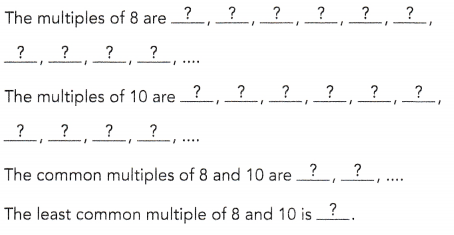Method 2
By prime factorization,
8 = 2 •10 = 2 •Least common multiple = 2 •=The least common multiple of 8 and 10 is.

Method 3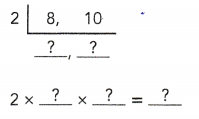The least common multiple of 8 and 10 is.

Find the least common multiple of each pair of numbers.

Question 19.
3 and 7
21

Explanation:
By prime factorization,
3 = 3, 7= 7
The least common multiple = 3 . 7 = 21
The least common multiple of 3 and 7 is 21.

Question 20.
5 and 12
60

Explanation:
By prime factorization,
5 = 5, 12 = 2 x 2 x 3
The least common multiple = 2 . 2 . 2. 3 . 5 = 60
The least common multiple of 5 and 12 is 60

Question 21.
4 and 9
36

Explanation:
By prime factorization,
4 = 2 x 2, 9 = 3 x 3
The least common multiple = 2 . 2 . 3 . 3 = 36
The least common multiple of 4 and 9 is 36

Question 22.
6 and 11
66

Explanation:
By prime factorization,
6 = 2 x 3, 11 = 11
The least common multiple = 2 . 3 . 11 = 66
The least common multiple of 6 and 11 is 66.

### Math in Focus Course 1A Practice 1.3 Answer Key

Find the common factors of each pair of numbers.

Question 1.
18 and 63
1, 3 and 9

Explanation:
The factors of 18 are 1, 2, 3, 6, 9, 18
The factors of 63 are 1, 3, 7, 9, 21, 63
The common factors of 18 and 63 are 1, 3, 9.

Question 2.
15 and 75
1, 3, 5 and 15.

Explanation:
The factors of 15 are 1, 3, 5, 15
The factors of 75 are 1, 3, 5, 15, 25, 75
The common factors of 15 and 75 are 1, 3, 5, 15.

Question 3.
30 and 50
1, 2, 5 and 10

Explanation:
The factors of 30 are 1, 2, 3, 5, 6, 10, 15, 30
The factors of 50 are 1, 2, 5, 10, 25, 50
The common factors of 30 and 500 are 1, 2, 5, 10.

Question 4.
64 and 92
1, 2 and 4.

Explanation:
The factors of 64 are 1, 2, 4, 8, 16, 32, 64
The factors of 92 are 1, 2, 4, 23, 46, 92
The common factors of 44 and 92 are 1, 2, 4.

Question 5.
26 and 78
1, 2, 13 and 26.

Explanation:
The factors of 26 are 1, 2, 13, 26
The factors of 78 are 1, 2, 6, 13, 26, 39, 78
The common factors of 26 and 78 are 1, 2, 13, 26.

Question 6.
55 and 88
1 and 11.

Explanation:
The factors of 55 are 1, 5, 11, 55
The factors of 88 are 1, 2, 4, 8, 11, 22, 44, 88
The common factors of 55 and 88 are 1, 11.

Find the greatest common factor of each pair of numbers.

Question 7.
24 and 36
12

Explanation:
The factors of 24 are 1, 2, 3, 4, 6, 8, 12, 24
The factors of 36 are 1, 2, 3, 4, 6, 9, 12, 18, 36
The common factors are 1, 2, 3, 4, 6, 12
The greatest common factors of 24 and 36 is 12.

Question 8.
30 and 54
6

Explanation:
The factors of 30 are 1, 2, 3, 5, 6, 10, 15, 30
The factors of 54 are 1, 2, 3, 6, 9, 18, 27, 54
The common factors are 1, 2, 3, 6
The greatest common factors of 30 and 54 is 6.

Question 9.
42 and 98
14

Explanation:
The factors of 42 are 1, 2, 3, 6, 7, 14, 21, 42
The factors of 98 are 1, 2, 7, 14, 49, 98
The common factors are 1, 2, 7, 14
The greatest common factors of 42 and 98 is 14.

Question 10.
48 and 72
24

Explanation:
The factors of 48 are 1, 2, 3, 4, 6, 8, 12, 16, 24, 48
The factors of 72 are 1, 2, 3, 4, 6, 8, 9, 12, 18, 24, 36, 72
The common factors are 1, 2, 3, 4, 6, 8, 12, 24
The greatest common factors of 48 and 72 is 24.

Question 11.
65 and 91
13

Explanation:
The factors of 65 are 1, 5, 13, 65
The factors of 91 are 1, 7, 13, 91
The common factors are 1, 13
The greatest common factors of 65 and 91 is 13.

Question 12.
84 and 100
4

Explanation:
The factors of 84 are 1, 2, 3, 4, 6, 7, 12, 14, 21, 28, 42, 84
The factors of 100 are 1, 2, 4, 5, 10, 20, 25, 50, 100
The common factors are 1, 2, 4
The greatest common factors of 84 and 100 is 4.

Find the first five common multiples of each pair of numbers.

Question 13.
5 and 6
30, 60, 90, 120 and 150

Explanation:
The multiples of 5 are 5, 10, 15, 20, 25, 30, 35, 40, 45, 50, 55, 60, 65…..
The multiples of 6 are 6, 12, 18, 24, 30, 36, 42, 48, 54, 60….
First five common multiples of 5 and 6 are 30, 60, 90, 120, 150.

Question 14.
4 and 7
28, 56, 84, 112, 140

Explanation:
The multiples of 4 are 4, 8, 12, 16, 20, 24, 28, 32, 36, 40, 44, 48, 52, 56, 60….
The multiples of 7 are 7, 14, 21, 28, 35, 42, 49, 56, 63, 70…..
First five common multiples of 4 and 7 are 28, 56, 84, 112 and 140

Question 15.
9 and 10
90, 180, 270, 360 and 450.

Explanation:
The multiples of 9 are 9, 18, 27, 36, 45, 54, 63, 72, 81, 90…..
The multiples of 10 are 10, 20, 30, 40, 50, 60, 70, 80 , 90…..
First five common multiples of 10 and 9 are 90, 180, 270, 360, 450.

Question 16.
8 and 11
88, 176, 264, 352 and 440.

Explanation:
The multiples of 8 are 8, 16, 24, 32, 40, 48, 56, 64, 72, 80, 88…
The multiples of 11 are 11, 22, 33, 44, 55, 66, 77, 88, 99, 110
First five common multiples of 8 and 11 are 88, 176, 264, 352 and 440.

Question 17.
15 and 25
75, 150 and 225.

Explanation:
The multiples of 15 are 15, 30, 45, 60, 75, 90, 105, 120, 135, 150….
The multiples of 25 are 25, 50, 75, 100, 125, 150, 175, 200, 225….
First five common multiples of 15 and 25 are 75, 150 and 225.

Question 18.
7 and 20
140, 280, 420, 560 and 700

Explanation:
The multiples of 7 are 7, 14, 21, 28, 35, 42, 49, 56, 63, 70…..
The multiples of 20 are 20, 40, 60, 80, 100, 120, 140,160…
First five common multiples of 7 and 20 are 140, 280, 420, 560 and 700.

Find the least common multiple of each pair of numbers.

Question 19.
3 and 10
30

Explanation:
By prime factorization,
3 = 3, 10 = 2 x 5
The least common multiple = 3 . 2 . 5 = 30
The least common multiple of 3 and 10 is 30.

Question 20.
7 and 12
84

Explanation:
By prime factorization,
7 = 7, 12 = 2 x 2 x 3
The least common multiple = 7 . 2 . 2 . 3
The least common multiple of 7 and 12 is 84.

Question 21.
5 and 8
40

Explanation:
By prime factorization,
5 = 5, 8= 2 x 2 x 2
The least common multiple = 5 . 2 . 2 . 2 = 40
The least common multiple of 5 and 8 is 40.

Question 22.
9 and 11
99

Explanation:
By prime factorization,
9 = 3 x 3, 11 = 11
The least common multiple = 3 . 3 .11 = 99
The least common multiple of 9 and 11 is 99.

Question 23.
10 and 14
70

Explanation:
By prime factorization,
10 = 2 x 5, 14 = 2. 7
The least common multiple = 2 . 5 . 7
The least common multiple of 10 and 14 is 70.

Question 24.
18 and 24
72

Explanation:
By prime factorization,
18 = 2 x 3 x 3, 24 = 2 x 2 x 2 x 3
The least common multiple = 2 . 2 . 2 . 3 . 3 = 72
The least common multiple of 18 and 24 is 72.

Find the greatest common factor of each set of numbers.

Question 25.
24, 26, and 84
2

Explanation:
The factors of 24 are 1, 2, 3, 4, 6, 8, 12, 24.
The factors of 26 are 1, 2, 13, 26
The factors of 84 are 1, 2, 4, 6, 7, 12, 14, 21, 28, 42, 84
The greatest common factor of 24, 26 and 84 is 2.

Question 26.
30, 48, and 72
6

Explanation:
The factors of 30 are 1, 2, 3, 5, 6, 10, 15, 30
The factors of 48 are 1, 2, 3, 4, 6, 8, 12, 16, 24, 48
The factors of 72 are 1, 2, 3, 4, 6, 8, 9, 12, 18, 24, 36, 72
The greatest common factor of 30, 48 and 72 is 6.

Question 27.
36, 24, and 96
12

Explanation:
The factors of 36 are 1, 2, 3, 4, 6, 9, 12, 18, 36
The factors of 24 are 1, 2, 3, 4, 6, 8, 12, 24
The factors of 96 are 1, 2, 3, 4, 6, 8, 12, 16, 24, 32, 48, 96
The greatest common factor of 36, 24 and 96 is 12.

Question 28.
42, 90, and 81
3

Explanation:
The factors of 42 are 1, 2, 3, 6, 7, 14, 21, 42
The factors of 90 are 1, 2, 3, 5, 6, 9, 10, 15, 18, 30, 45, 90
The factors of 81 are 1, 3, 9, 27, 81
The greatest common factor of 42, 90 and 81 is 3

Question 29.
60, 75, and 102
3

Explanation:
The factors of 60 are 1, 2, 3, 4, 5, 6, 10, 12,15, 20, 30, 60
The factors of 75 are 1, 3, 5, 15, 25, 75
The factors of 102 are 1, 2, 3, 6, 17, 34, 51, 102
The greatest common factor of 60, 75 and 102 is 3.

Question 30.
63, 105, and 294
7

Explanation:
The factors of 63 are 1, 3, 7, 9, 21, 63
The factors of 105 are 1, 3, 5, 7, 15, 21, 35, 105
The factors of 294 are 1, 2, 3, 6, 7, 14, 21, 42, 49, 98, 147, 294
The greatest common factor of 63, 105 and 294 is 7.

Find the least common multiple of each set of numbers.

Question 31.
18, 24, and 42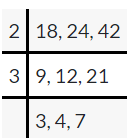Explanation:
The least common multiple of 18, 24 and 42 is 2 x 3 x 3 x 4 x 7 = 504.

Question 32.
21, 33, and 57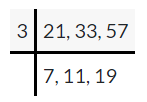Explanation:
The least common multiple of 21, 33 and 57 is 3 x 7 x 11 x 19 = 4389.

Question 33.
14, 30, and 70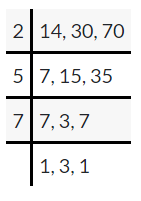Explanation:
The least common multiple of 14, 30 and 70 is 2 x 5 x 7 x 3 = 210.

Question 34.
27, 48, and 66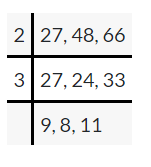Explanation:
The least common multiple of 27, 48 and 66 is 2 x 3 x 9 x 8 x 11 = 4752.

Question 35.
55, 75, and 115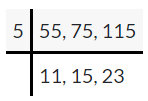Explanation:
The least common multiple of 55, 75 and 115 is 5 x 11 x 15 x 23 = 18975.

Question 36.
78, 90, and 140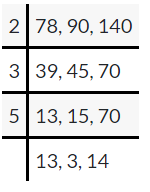Explanation:
The least common multiple of 78, 90 and 140 is 2 x 3 x 3 x 5 x 13 x 14 = 16380.

Find the greatest common factor and the least common multiple of each set of numbers.

Question 37.
10, 20, and 25
5, 100

Explanation:
By prime factorization,
10 = 2 x 5, 20 = 2 x 2 x 5, 25 = 5 x 5
The least common multiple = 2 . 5 . 2 . 5 = 100
The least common multiple of 10 , 20 and 25 is 100.
The greatest common factor is 5.

Question 38.
16, 28, and 40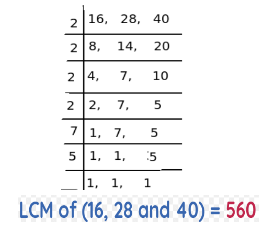Greatest common factor is 4.

Explanation:
The least common multiple of 16, 28 and 135 is 560.
By prime factorization:
16 = 2 x 2 x 2 x 2, 28 = 2 x 2 x 7, 40 = 2 x 2 x 2 x 5
common factors are 2 x 2
The greatest common factor is 4.

Question 39.
54, 81, and 135
27, 810

Explanation:
By prime factorization,
54 = 2 x 3 x 3 x 3, 81= 3 x 3 x 3 x 3, 135 = 3 x 3 x 3 x 5
The least common multiple =2 . 3 . 3 . 3 . 3  . 5
The least common multiple of 84 , 81 and 135 is 810
The greatest common factor is 3 x 3 x 3 = 27.

Question 40.
72, 144, and 216
72, 432

Explanation:
By prime factorization,
72 = 2 x 2 x 2 x 3 x 3, 144 = 2 x 2 x 2 x 2 x 3 x 3, 216 = 2 x 2 x 2 x 3 x 3 x 3
The least common multiple = 2 . 2 . 2 . 2 . 3 . 3 . 3
The least common multiple of 72 , 114 and 216 is 432.
The greatest common factor is 2 x 2 x 2 x 3 x 3 = 72

Solve.

Question 41.
Makayla has two types of ropes. She wants to cut the ropes into pieces of the same length for butterfly knots,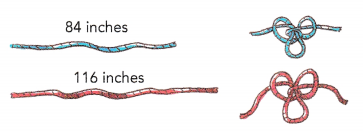a) Find the greatest possible length that she can cut for each piece, so that no rope will be left unused.

b) Write the sum of the two lengths and factor out the number you found in part a). What does the number inside the parentheses represent?

Question 42.
Giselle buys two types of flowers, 48 pink roses and 56 white lilies. She combines the flowers to make identical bouquets, with no flowers left over.
a) Find the greatest number of bouquets that Giselle can make.
Giselle can make 8 bouquets

Explanation:
By prime factorization,
48 = 2 x 2 x 2 x 2 x 3, 56 = 2 x 2 x 2 x 7
common multiple is 2 x 2 x 2 = 8
Greatest common multiple of 48  and 56 is 8

b) Find the number of pink roses and white lilies in each bouquet.
6 pink roses and 7 white lilies

Explanation:
pink roses = 48 = 8 x 6
white lilies = 56 = 8 x 7
So, Each bouquet has 6 pink roses and 7 white lilies.

Question 43.
A red light flashes every 14 minutes. A blue light flashes every 24 minutes. When will the two lights flash together again, if they last flashed together at 8 A.M.?

Question 44.
a) Find the product of 84 and 90.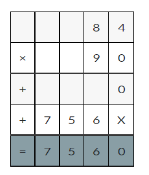Explanation:
The product of 84 and 90 is 7560.

b) Find the product of the greatest common factor and the least common multiple of 84 and 90.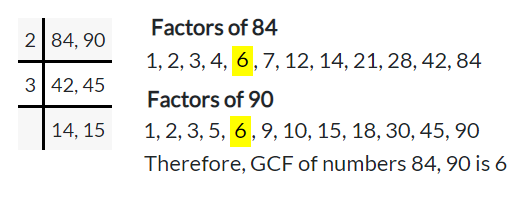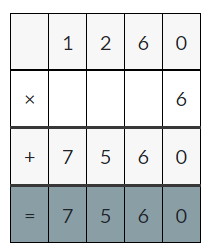Explanation:
Least common multiple of 84 and 90 is 1260,
Greatest common factor of 84 and 90 is 6
Product of the greatest common factor and the least common multiple of 84 and 90 is
1260 x 6 = 7560.

c) What do you observe about your answers to parts a) and b)?